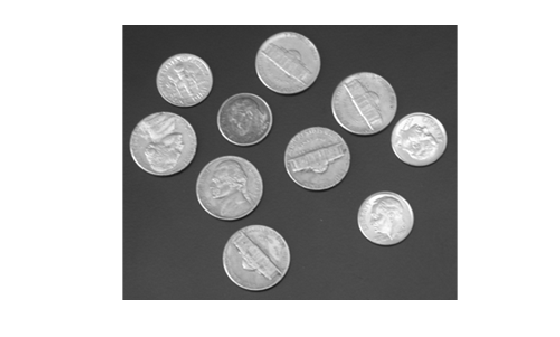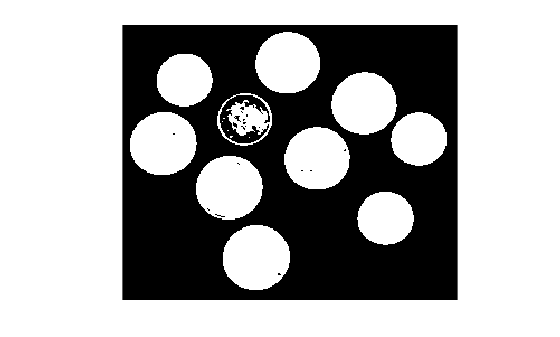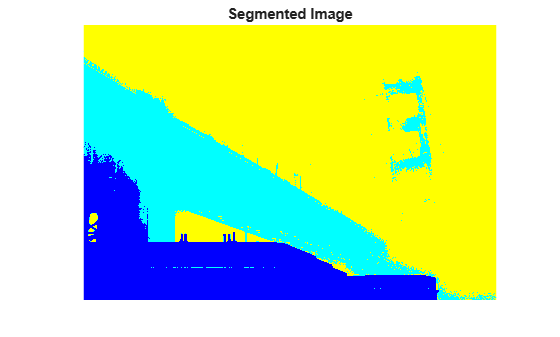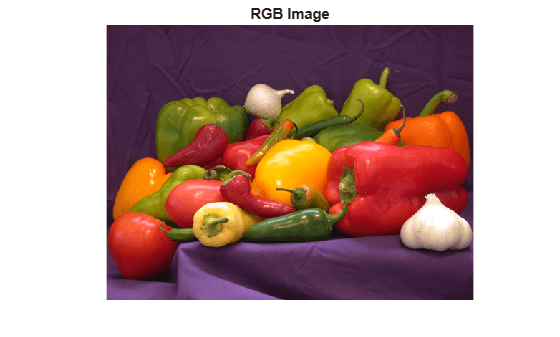Documentation

# multithresh

Multilevel image thresholds using Otsu’s method

## Syntax

``thresh = multithresh(A)``
``thresh = multithresh(A,N)``
``````[thresh,metric] = multithresh(___)``````

## Description

example

````thresh = multithresh(A)` returns the single threshold value `thresh` computed for image `A` using Otsu’s method. You can use `thresh` as an input argument to `imquantize` to convert an image into a two-level image.```

example

````thresh = multithresh(A,N)` returns `thresh` a 1-by-N vector containing `N` threshold values using Otsu’s method. You can use `thresh` as an input argument to `imquantize` to convert image `A` into an image with `N + 1` discrete levels.```

example

``````[thresh,metric] = multithresh(___)``` returns `metric`, a measure of the effectiveness of the computed thresholds. `metric` is in the range `[0 1]` and a higher value indicates greater effectiveness of the thresholds in separating the input image into `N + 1` regions based on Otsu's objective criterion.```

## Examples

collapse all

Read image and display it.

```I = imread('coins.png'); imshow(I)```Calculate a single threshold value for the image.

`level = multithresh(I);`

Segment the image into two regions using `imquantize` , specifying the threshold level returned by `multithresh` .

```seg_I = imquantize(I,level); figure imshow(seg_I,[])```Read image and display it.

```I = imread('circlesBrightDark.png'); imshow(I) axis off title('Original Image')```Calculate two threshold levels.

`thresh = multithresh(I,2);`

Segment the image into three levels using `imquantize` .

`seg_I = imquantize(I,thresh);`

Convert segmented image into color image using `label2rgb` and display it.

```RGB = label2rgb(seg_I); figure; imshow(RGB) axis off title('RGB Segmented Image')```Read truecolor (RGB) image and display it.

```I = imread('peppers.png'); imshow(I) axis off title('RGB Image');```Generate thresholds for seven levels from the entire RGB image.

`threshRGB = multithresh(I,7);`

Generate thresholds for each plane of the RGB image.

```threshForPlanes = zeros(3,7); for i = 1:3 threshForPlanes(i,:) = multithresh(I(:,:,i),7); end```

Process the entire image with the set of threshold values computed from entire image.

```value = [0 threshRGB(2:end) 255]; quantRGB = imquantize(I, threshRGB, value);```

Process each RGB plane separately using the threshold vector computed from the given plane. Quantize each RGB plane using threshold vector generated for that plane.

```quantPlane = zeros( size(I) ); for i = 1:3 value = [0 threshForPlanes(i,2:end) 255]; quantPlane(:,:,i) = imquantize(I(:,:,i),threshForPlanes(i,:),value); end quantPlane = uint8(quantPlane);```

Display both posterized images and note the visual differences in the two thresholding schemes.

```imshowpair(quantRGB,quantPlane,'montage') axis off title('Full RGB Image Quantization Plane-by-Plane Quantization')```To compare the results, calculate the number of unique RGB pixel vectors in each output image. Note that the plane-by-plane thresholding scheme yields about 23% more colors than the full RGB image scheme.

```dim = size( quantRGB ); quantRGBmx3 = reshape(quantRGB, prod(dim(1:2)), 3); quantPlanemx3 = reshape(quantPlane, prod(dim(1:2)), 3); colorsRGB = unique(quantRGBmx3, 'rows' ); colorsPlane = unique(quantPlanemx3, 'rows' ); disp(['Unique colors in RGB image : ' int2str(length(colorsRGB))]);```
```Unique colors in RGB image : 188 ```
`disp(['Unique colors in Plane-by-Plane image : ' int2str(length(colorsPlane))]);`
```Unique colors in Plane-by-Plane image : 231 ```

`I = imread('circlesBrightDark.png');`

Find all unique grayscale values in image.

```uniqLevels = unique(I(:)); disp(['Number of unique levels = ' int2str( length(uniqLevels) )]);```
```Number of unique levels = 148 ```

Compute a series of thresholds at monotonically increasing values of `N`.

```Nvals = [1 2 4 8]; for i = 1:length(Nvals) [thresh, metric] = multithresh(I, Nvals(i) ); disp(['N = ' int2str(Nvals(i)) ' | metric = ' num2str(metric)]); end```
```N = 1 | metric = 0.54767 N = 2 | metric = 0.98715 N = 4 | metric = 0.99648 N = 8 | metric = 0.99902 ```

Apply the set of 8 threshold values to obtain a 9-level segmentation using `imquantize` .

```seg_Neq8 = imquantize(I,thresh); uniqLevels = unique( seg_Neq8(:) )```
```uniqLevels = 9×1 1 2 3 4 5 6 7 8 9 ```

Threshold the image using `seg_Neq8` as an input to `multithresh`. Set `N` equal to 8, which is 1 less than the number of levels in this segmented image. `multithresh` returns a `metric` value of 1.

`[thresh, metric] = multithresh(seg_Neq8,8)`
```thresh = 1×8 1.8784 2.7882 3.6667 4.5451 5.4549 6.3333 7.2118 8.1216 ```
```metric = 1 ```

Threshold the image again, this time increasing the value of `N` by 1. This value now equals the number of levels in the image. Note how the input is degenerate because the number of levels in the image is too few for the number of requested thresholds. Hence, multithresh returns a `metric` value of 0.

`[thresh, metric] = multithresh(seg_Neq8,9)`
```Warning: No solution exists because the number of unique levels in the image are too few to find 9 thresholds. Returning an arbitrarily chosen solution. ```
```thresh = 1×9 1 2 3 4 5 6 7 8 9 ```
```metric = 0 ```

## Input Arguments

collapse all

Image to be thresholded, specified as a real, nonsparse numeric array of any dimension. `multithresh` finds the thresholds based on the aggregate histogram of the entire array. `multithresh` considers an RGB image as a 3-D numeric array and computes the thresholds for the combined data from all three color planes.

`multithresh` uses the range of the input image `A`, `[min(A(:)) max(A(:))]`, as the limits for computing the histogram used in subsequent computations. `multithresh` ignores any `NaNs` in computation. Any `Infs` and `-Infs` are counted in the first and last bin of the histogram, respectively.

For degenerate inputs where the number of unique values in `A` is less than or equal to `N`, there is no viable solution using Otsu's method. For such inputs, the return value `thresh` contains all the unique values from `A` and possibly some extra values that are chosen arbitrarily.

Example: ```I = imread('cameraman.tif'); thresh = multithresh(I);```

Data Types: `single` | `double` | `int16` | `uint8` | `uint16`

Number of threshold values, specified as a positive integer scalar value. For `N > 2`, `multithresh` uses search-based optimization of Otsu's criterion to find the thresholds. The search-based optimization guarantees only locally optimal results. Since the chance of converging to local optimum increases with `N`, it is preferable to use smaller values of `N`, typically ```N < 10```. The maximum allowed value for `N` is 20.

Example: `thresh = multithresh(I,4);`

Data Types: `single` | `double` | `int8` | `int16` | `int32` | `int64` | `uint8` | `uint16` | `uint32` | `uint64`

## Output Arguments

collapse all

Set of threshold values used to quantize an image, returned as a 1-by-N vector, whose data type is the same as image `A`.

These thresholds are in the same range as the input image `A`, unlike the `graythresh` function, which returns a normalized threshold in the range `[0, 1]`.

Measure of the effectiveness of the thresholds, returned as a scalar value. Higher values indicates greater effectiveness of the thresholds in separating the input image into `N+1` classes based on Otsu's objective criterion. For degenerate inputs where the number of unique values in `A` is less than or equal to `N`, `metric` equals 0.

Data Types: `double`

 Otsu, N., "A Threshold Selection Method from Gray-Level Histograms," IEEE Transactions on Systems, Man, and Cybernetics, Vol. 9, No. 1, 1979, pp. 62-66.